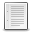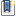File : Details

# partfrac()

Partial fraction decomposition of a rational in the C set
Details
Version
1.1
A more recent valid version with binaries for Scilab exists: 1.3.1
Author
Samuel Gougeon
Maintainer
Samuel Gougeon
Category
Supported Scilab Version
5.5
Creation Date
August 27, 2015Description
```            --> partfrac

Partial fraction decomposition of a rational in the C set

SYNTAX
------
partfrac    // Displays this help
i       = partfrac(f)
[i,r]    = partfrac(f)
[i,r,d]  = partfrac(f)
[i,r,d,t]= partfrac(f)

PARAMETERS
----------
f : Single rational = polynomial fraction with real or complex coefficients.
i : Integer part = non-fractional part of f (single polynomial).
r : Remainder of f: rational with degree(numerator) < degree(denominator)

d : (3xN) matrix describing the N terms of the r's decomposition:
d(1,:): real or complex coefficient of numerators.
d(2,:): real or complex value of the considered poles.
d(3,:): integer>0: powers m of the denominators (x-pole)^m
d is such that sum( d(1,:)./ ((x-d(2,:)).^real(d(3,:))) ) - r == 0.
NOTE: real() must be applied to d(3,:) only because d is complex-encoded.
See http://bugzilla.scilab.org/14116

t : 3-rows column of Texts. write(%io(2),t) displays i+d in a comprehensive
way. The format of coefficients is set with format().

DESCRIPTION
-----------
From a single polynomial fraction f = p/q with coprime polynomials p and q,
partfrac(f) extracts
* the non fractional part i of f: polynomial such that
0 <= degree(i) <= degree(p) - degree(q): i = p - modulo(p,q)
* the fractional part of f, or remainder r of p/q : r = modulo(p,q)/q
such that degree(numer(r))< degree(q) and  f = i + r
* the rational decomposition of r, aka partial fraction decomposition of f:
The 'vector' of elementary rationals c/(x-pole)^m where c and poles are
decimal or complex numbers and m are the multiplicities of poles:
c = d(1,:), pole = d(2,:), m = d(3,:)

In addition, partfrac() may return as text the literal expression t of the
whole decomposition of f. This form shows the factorized forms of denominators
where poles that are multiple appear with their powers. write(%io(2), t) may
be used to display it.

DEPENDENCY: If d or/and t is expected, polyroots() is required (> See also)

NOTE: pfss(f) yields a list mixing the non-fractional part (as last element)
with the decomposition, and where terms of the decomposition are not always
elementary: their denominator may be of order 2 even for real poles. See the
example.

FEEDBACK
---------
Comments, scoring and bug reports are welcome on
http://fileexchange.scilab.org/toolboxes/451000#new_comment

--------
pfss      : partial (uncomplete) fraction decomposition of a linear system
modulo    : remainder after polynomial division
polyroots : Multiplicities and values of polynomial multiple roots:
http://fileexchange.scilab.org/toolboxes/362000
pdiv_inc  : Polynomial division with increasing terms powers:
http://fileexchange.scilab.org/toolboxes/449000

EXAMPLE
-------
x = poly(0,"x");
f = (3-x+x^2-4*x^3+2*x^4) / ((x-1)*(x-2)^2)
[E, R, F, T] = partfrac(f);
E, R, F, T
D = F(1,:)./((x-F(2,:)).^real(F(3,:)))
clean(R-sum(D))
// Comparison with pfss():
list2vec(pfss(f)).'

RESULTS
-------

-->x = poly(0,"x");

--> f = (3-x+x^2-4*x^3+2*x^4) / ((x-1)*(x-2)^2)
f  =
2    3    4
3 - x + x - 4x + 2x
-------------------
2   3
- 4 + 8x - 5x + x

--> [E, R, F, T] = partfrac(f);

--> E,R,F,T
E  =
6 + 2x

R  =
2
27 - 41x + 15x
--------------
2   3
- 4 + 8x - 5x + x

F  =
14.    5.    1.
2.     2.    1.
1.     2.    1.

T  =
!          14       5        1    !
!6 + 2x + ---- + -------- + ----  !
!         -2+x   (-2+x)^2   -1+x  !

--> D = F(1,:)./((x-F(2,:)).^real(F(3,:)))

D  =
!     14            5             1     !
!  ---------  --------------  --------- !
!                          2            !
!  -2 +  1x    4 - 4x +  1x   -1 +  1x  !

--> clean(R-sum(D))
ans  =
0
-
1

--> // Comparison with pfss():

--> list2vec(pfss(f)).'
ans  =

- 23 + 14x        1       6 + 2x
----------     -----     ------
2
4 - 4x + x   - 1 + x       1
```Files (1)Miscellaneous file
```File defining the function partfrac(), help contents and examples

* MAKE partfrac() AVAILABLE in EVERY SESSION: Click here to see HOW TO

* Get help: Enter "partfrac" without parameters

CHANGES 1.0 => 2.0
------------------
* The format of the returned decomposition was a vector of rationals.
It is now a 3-row matrix specifying for each term, the numerator
(coefficient), the value of the considered pole, and the 'pole exponent'.News (0)Comments (0)Leave a comment
Login withEmail notifications